# Types of Basic Sets

In the previous article, you learnt about set notations – the roster notation and the set builder notation. In this article, I will discuss about the types of sets available. This is part 1 where we see basic types of sets and in part 2, I will discuss some derived types.

## Set Types

The sets are collections of objects with some properties, but not all sets are same. Mathematicians have identified that certain sets have special properties and named them accordingly.

We have different type of sets, listed here.

1. equal set
2. equivalent set
3. empty set
4. singleton set

let us understand each of these set type. Also, I am giving examples of each set type so that it is easy to understand. For more examples, you can refer to some math books.

### Equal Sets

We can define the equal set informally as

” Two sets are equal if and only if they have the same elements.”

If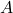andare two equal sets, elements are setare inand elements of setis in set. It meansandare equal sets, if and only if

\begin{aligned} \forall x ( x \in A \iff x \in B) \end{aligned}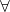(For all) is a universal quantifier, which is considering all elements from a set or universe. The symbol (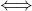) is biconditional, meaning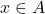is only true if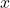is also inoris only true whenis in.

To represent an equal set, we write

A = B

For example, Letbe set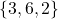.

A = \{3, 6, 2\}

andbe set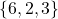.

B = \{6, 2, 3\}

Bothandare equal (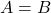), since, they have the same elements. Here order of elements does not matter.

### Equivalent sets

The equivalent set is defined as:

“Two or more set are equivalent if their cardinality is same”.

Here cardinality is simply meaning number of elements and shown as a function of set.

Therefore,

Ifandare two sets then they are equivalent if and only if

n(A) = n(B)

For example, let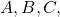and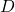are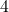sets.

\begin{aligned}
&A = \{ 3, 1, 7\}\\
&B = \{p, q , r\}\\
&C = {4, 3, 2, 8, 9}\\
&D = {1, x, 3, y, 8}
\end{aligned}

The elements of setare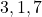which are numbers. and elements of setare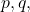and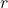which is alphabets. The elements of set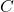are numbers and elements of setare both numbers and alphabets.

First, we determine the cardinality of sets –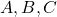andusing the cardinality function.

\begin{aligned}
&n(A) = 3 \\
&n(B) = 3 \\
&n(C) = 5 \\
&n(D) = 5 \\
\end{aligned}

The cardinality of setand setis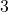, so they are equivalent sets. The cardinality of setand Setis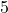, so they are another equivalent set.
Note that the elements don’t have to be same. Set with different types of elements can be equivalent.

### Comparison between equal and Equivalent set

Let us compare between equal sets and equivalent sets.

• Two or sets have same elements, so they can be equal and equivalent at the same time.
• For equivalent sets, the elements does not matter, only cardinality is important, whereas equal sets consider elements also.
• order of elements in both equal and equivalent sets does not matter.
• empty sets are always equivalent to each other.

When two sets have same element, they are equal set which we know already, but they have the same cardinality also. For instance,

\begin{aligned}
&A = \{2, 5 , 7\}\\
&B = \{5, 2, 7\}
\end{aligned}

The setandare same, hence, equal sets. If we check the cardinality of both sets, then

n(A) = 3\\n(B) = 3

It means that the number of elements is also same for both functions.

Another thing to notice in setand setis that order of elements is different. The order of elements is does not matter in equal sets. Also, it does matter how the elements are arranged when we are only concerned with number of elements of the set. Therefore, equivalent sets are also not affected by the order of elements in a set.

### Empty sets

The empty sets are sets with no elements. They are denoted with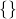or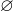. Sometimes a set is described in terms of properties. For example,

Set of months in a year.

If the set property is such that there is no element exists is also empty.

For example,

A set of numbers with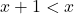, it is not possible, so such a set contains no element, and it is an empty set. It is also called the null set.

The empty set is this symboland this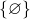is a set that contains empty set.

### Singleton Set

A singleton set is a set with only one element.

For example,

Set of even prime,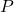is only one element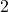.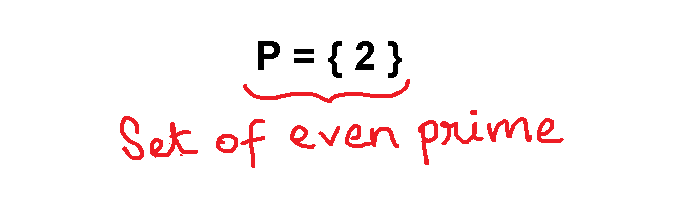Figure 1 – Set of even prime is a property with only one element which is 2.

Other examples of singleton sets are :

\begin{aligned}
&Set \hspace{3px} A = \{ 0 \}\\
&or \\
&Set \hspace{3px} B = \{ x \in \mathbb{Z} \mid x + 4 = 0 \}
\end{aligned}


Wherecan be only one number which is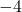in set.

## Finite Sets

All of the previous set types have one thing in common, which is, the number of elements is limited or countable. A set with limited element is called a finite set. There is no special symbol to denote a finite set.

But, when you use roster notation, then you can list all the elements of the set. In other words, roster method is most suitable way to show a finite set.

For example, the set of all number less than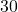perfectly divisible by numberare:

\begin{aligned}
S = \{ 5, 10, 15, 20, 25 \}
\end{aligned}

Note, how set builder notion is very descriptive and long while describing a set of finite elements. That’s why a roster method is best choice for represent finite sets.

What happens when the set has infinite number of elements ?

## Infinite Sets

A set with infinite number of elements is known as infinite set. It this set elements are many, to roster method is not a suitable method to represent the set.

Consider an example,

N = \{Set \hspace{3px} of \hspace {3px} natural \hspace{ 3px} numbers \}

The natural numbers are infinite and uncountable set. If that is the case, then describing the natural number set using set builder notation is suitable option.

It does not mean that infinite sets cannot be showed as using roster notation. But you must use the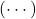symbol to show that there are infinite number of elements in the set.

Therefore, the set of natural numbers can be represented as:

N = \{ 1, 2, 3, 4, \cdots \}

The set of integers grows continuously on both direction, which is negative and positive. Therefore, it is shown as:

Z = \{ \cdots, -3, -2, -1 , 0, 1, 2, 3 , \cdots \}

There are several examples of infinite sets in mathematics. You will learn those in future articles.

## Summary

You learnt that the sets are broadly finite or infinite sets. Depending on element in the sets. So a two sets can be equal or equivalent depending on number and type of elements in them. There are empty or singleton sets with no or one element respectively.